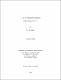## A corona theorem for certain subalgebras of H∞(D)2009
##### Publisher
University of Alabama Libraries
##### Abstract

[NOTE: Text or symbols not renderable in plain text are indicated by [...]. See PDF document for full abstract.] The corona theorem for the space of bounded analytic functions on the unit disk, [...], which was proven by Carleson in 1962, states that D is dense in the maximal ideal space of [...]. This theorem can be reduced to the following result: [...]. Furthermore, if we have the additional condition that [...]. In this dissertation, we prove that the corona theorem holds for certain subalgebras of [...], and we provide estimates for the sizes of the given solutions. Among the algebras we consider are those which contain bounded analytic functions whose kth derivatives vanish at 0 for all k in K, a subset of the natural numbers, which we call [...]. We give several properties the set K must have in order for [...] to be an algebra. We then prove the corona theorem in both the vector and matrix cases for these algebras. In fact, in the vector case, we prove the corona theorem using two different techniques. Each gives a unique estimate, and one extends our findings to more general algebras. We also settle a conjecture of Mortini, Sasane, and Wick involving the algebra C+BH∞(D), where B is a Blaschke product. We prove the corona theorem in C+BH∞(D) holds for an infinite number of functions. We end with a few suggestions for future research.

##### Description
Electronic Thesis or Dissertation
Mathematics